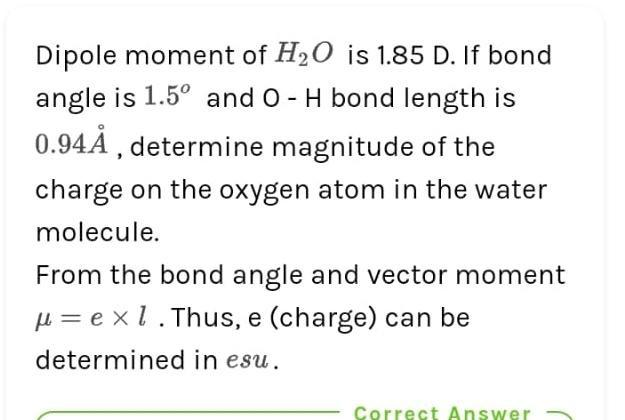Question:

# Dipole moment of H2O is 1.85 D. If bond angle is 1.5º and O-H bond length is 0.94Å , determine magnitude of the charge on the oxDipole moment of H2O is 1.85 D. If bond angle is 1.5º and O-H bond length is 0.94Å , determine magnitude of the charge on the oxygen atom in the water molecule. From the bond angle and vector moment u= exl. Thus, e (charge) can be determined in esu. Correct Answer